﻿ The Legendre Polynomials Associated with Bernoulli, Euler, Hermite and Bernstein Polynomials

### The Legendre Polynomials Associated with Bernoulli, Euler, Hermite and Bernstein Polynomials

Serkan Araci, Mehmet Acikgoz, Armen Bagdasaryan, Erdoğan ŞenOPEN ACCESSPEER-REVIEWED

## The Legendre Polynomials Associated with Bernoulli, Euler, Hermite and Bernstein Polynomials

Serkan Araci1,, Mehmet Acikgoz2, Armen Bagdasaryan3, Erdoğan Şen4

1Atatürk Street, 31290 Hatay, Turkey

2University of Gaziantep, Faculty of Science and Arts, Department of Mathematics, Gaziantep, Turkey

3Russian Academy of Sciences, Institute for Control Sciences, Profsoyuznaya, Moscow, Russia

4Department of Mathematics, Faculty of Science and Letters, Namik Kemal University, Tekirdağ, Turkey

### Abstract

In the present paper, we deal mainly with arithmetic properties of Legendre polynomials by using their orthogonality property. We show that Legendre polynomials are proportional with Bernoulli, Euler, Hermite and Bernstein polynomials.

• Araci, Serkan, et al. "The Legendre Polynomials Associated with Bernoulli, Euler, Hermite and Bernstein Polynomials." Turkish Journal of Analysis and Number Theory 1.1 (2013): 1-3.
• Araci, S. , Acikgoz, M. , Bagdasaryan, A. , & Şen, E. (2013). The Legendre Polynomials Associated with Bernoulli, Euler, Hermite and Bernstein Polynomials. Turkish Journal of Analysis and Number Theory, 1(1), 1-3.
• Araci, Serkan, Mehmet Acikgoz, Armen Bagdasaryan, and Erdoğan Şen. "The Legendre Polynomials Associated with Bernoulli, Euler, Hermite and Bernstein Polynomials." Turkish Journal of Analysis and Number Theory 1, no. 1 (2013): 1-3.

 Import into BibTeX Import into EndNote Import into RefMan Import into RefWorks

### 1. Introduction

Legendre polynomials, which are special cases of Legendre functions, are introduced in 1784 by the French mathematician A. M. Legendre (1752-1833). Legendre functions are a vital and important in problems including spherical coordinates. Due to their orthogonality properties they are also useful in numerical analysis (see ). Besides, the Legendre polynomials,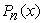, are described via the following generating function:(1)

Legendre polynomials are the everywhere regular solutions of Legendre’s differential equation that we can write as follows:where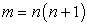and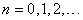. Taking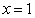in (1) and by using geometric series, we see that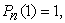so that the Legendre polynomials are normalized.

Legendre polynomials can be generated using Rodrigue’s formula as follows: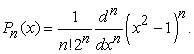(2)

Note that the right hand side of (2) is a polynomial (see [3, 9]).

The Bernoulli polynomials are defined by means of the following generating function:(3)

By (3), we know thatTakingin (3), we havethat stands for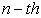Bernoulli number.

The Euler polynomials are known to be defined as: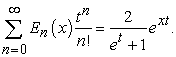(4)

The Euler polynomials can also be expressed by explicit formulas, e.g.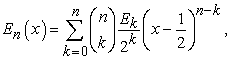where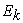means the Euler numbers. These numbers are expressed with the Euler polynomials throughNow also, we give the definition of Hermite polynomials as follows:(5)

Let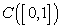be the space of continuous functions on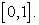ForBernstein operator foris defined by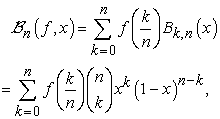whereandis the set of natural numbers. Hereis called Bernstein polynomials, which are defined by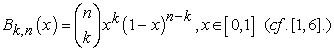(6)

In , , the orthogonality of Legendre polynomials is known as(7)

where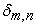is Kronecker’s delta.

In , by using orthogonality property of Legendre, Kim et al. effected interesting identities for them. We also obtain some interesting properties of the Legendre polynomials arising from Bernoulli, Euler, Hermite and Bernstein polynomials.

### 2. Identities on the Legendre Polynomials Arising from Bernoulli, Euler, Hermite and Bernstein Polynomials

LetThen we define an inner product on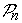as follows:(8)

Note thatare the orthogonal basis for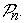Let us now considerthen we see that(9)

where the coefficients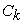are defined over the field of real numbers.

From the above, we readily see that(10)

By (9) and (10), we have the following proposition.

Proposition 2.1. Letand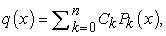then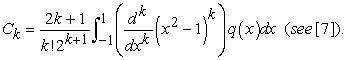If we takein Proposition (2.1), the coefficientscan be found as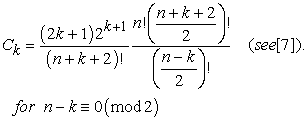(11)

LetThen by using Proposition 2.1 and (11), we have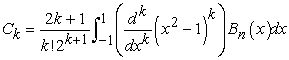where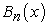are the aforementioned Bernoulli polynomials that can be expressed through Bernoulli numbers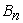as follows: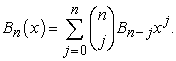From this, we have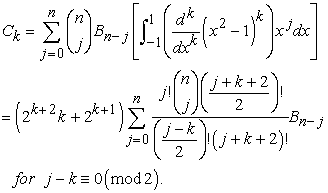Therefore we have the following theorem.

Theorem 2.2. Let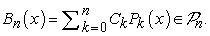Then we have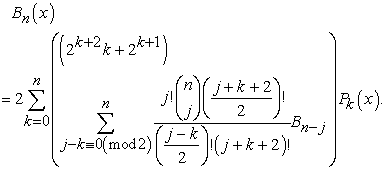LetBy Proposition 2.1 and (11), we have the following theorem.

Theorem 2.3. Let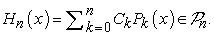Then we have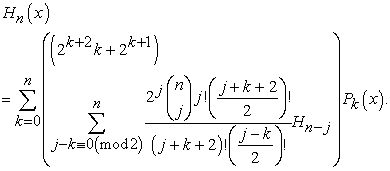Let the Bernstein polynomialsBy Proposition 2.1 and (11), we have following theorem.

Theorem 2.4. Let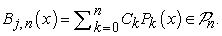We have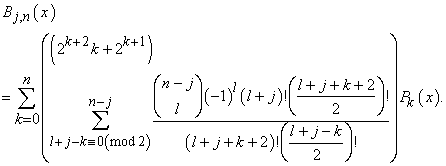The following equality is defined by Kim et al. in :(12)

Let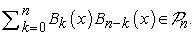By Proposition 2.1 and (11), we get the following theorem.

Theorem 2.5. LetThen we have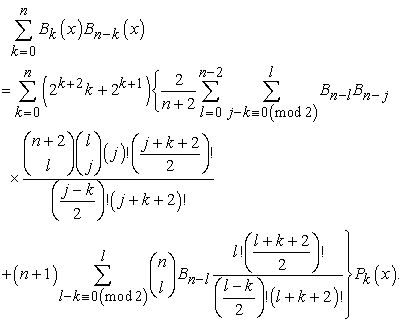LetIn , Kim et al. derived convolution formula for the Euler polynomials asBy Proposition 2.1 and (11), we get the following theorem.

Theorem 2.6. The following equality holds true:Remark 2.7. By using Theorem 2.1, we can find many interesting identities for the special polynomials in connection with Legendre polynomials.

### References

  S. Araci, D. Erdal and J. J. Seo, A study on the fermionic p-adic q-integral representation on P associated with weighted q-Bernstein and q-Genocchi polynomials, Abstract and Applied Analysis, Volume 2011 (2011), Article ID 649248, 10 pages.In article  A. Bagdasaryan, An elementary and real approach to values of the Riemann zeta function, Phys. Atom. Nucl. 73, 251-254, (2010).In article CrossRef  W. N. Bailey, On the product of two Legendre polynomials, Proc. Cambridge Philos. Soc. 29 (1933), 173-177.In article CrossRef  B. C. Kellner, On irregular prime power divisors of the Bernoulli numbers, Mathematics of Computation, Volume 76, Number 257, January 2007, Pages 405-441.In article CrossRef  T. Kim, Some identities on the q-Euler polynomials of higher order and q-stirling numbers by the fermionic p-adic integral on p, Russian J. Math. Phys. 16 (2009), 484-491.In article CrossRef  T. Kim, J. Choi, Y. H. Kim and C. S. Ryoo, On q-Bernstein and q-Hermite polynomials, Proc. Jangjeon Math. Soc. 14 (2011), no. 2, 215-221.In article  D. S. Kim, S.-H. Rim and T. Kim, Some identities on Bernoulli and Euler polynomials arising from orthogonality of Legendre polynomials, Journal of Inequalities and Applications 2012, 2012:227In article  D. S. Kim, T. Kim, S.-H. Lee, Y.-H. Kim, Some identities for the product of two Bernoulli and Euler polynomials. Adv. Diff. Equ. 2012; 2012:95.In article  L. C. Andrews, Special Functions of Mathematics for Engineerings, SPIE Press, 1992, pages 479.In article